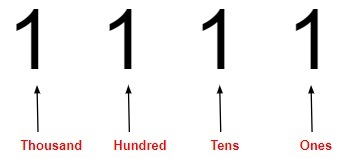# C Program to convert a given number to words

Given a string consisting of numerical values, the task is to covert those given numbers in words.

Like we have an input “361”; then the output should be in words i.e,” Three hundred sixty one”. For the solution of the following problem we have to keep in mind the numbers and places it is in on like ones, tens, thousands etc.

The code only support upto 4 digits numbers i.e., 0 to 9999. So the input should be from 0 to 9999.

Let us consider 1,111 so the places will be like −## Example

Input: “1234”
Output: one thousand two hundred thirty four
Input: “7777”
Output: seven thousand seven hundred seventy seven

Approach we will be using to solve the given problem

• Take the input as a string.
• Making arrays for different values.
• Checking the length of the input according to the length we will decide that till what places we will be showing output.
• According to the places will show the output.

## Algorithm

Start
Step 1 → In function convert(char *num)
Declare and initialize int len = strlen(num)
If len == 0 then,
fprintf(stderr, "empty string")
Return
End If
If len > 4 then,
fprintf(stderr, "Length more than 4 is not supported")
Return
End If
Declare and initialize a char *single_digit[] = { "zero", "one", "two","three", "four","five","six", "seven", "eight", "nine"}
Declare and initialize a char *tens_place[] = {"", "ten", "eleven", "twelve","thirteen", "fourteen","fifteen", "sixteen","seventeen", "eighteen", "nineteen"}
Declare and Initialize a char *tens_multiple[] = {"", "", "twenty", "thirty", "forty", "fifty","sixty", "seventy", "eighty", "ninety"}
Declare and initialize char *tens_power[] = {"hundred", "thousand"}
Print num
If len == 1 then,
Print single_digit[*num - '0']
Return
End If
While *num != '\0
If len >= 3
If *num -'0' != 0
Print single_digit[*num - '0']
Print tens_power[len-3]
End If
Decrement len by 1
End If
Else
If *num == '1' then,
Set sum = *num - '0' + *(num + 1)- '0'
Print tens_place[sum]
Return
End If
Else If *num == '2' && *(num + 1) == '0' then,
Print “twenty”
Return
End else If
Else
Set i = *num - '0'
Print i? tens_multiple[i]: ""
Increment num by 1
If *num != '0' then,
Print single_digit[*num - '0']
End If
End Else
Increment num by 1
End Else
End while
Step 2 → In function main()
Call function convert("9132")
Stop

## Example

#include <stdio.h>
#include <string.h>
#include <stdlib.h>
//function to print the given number in words
void convert(char *num) {
int len = strlen(num);
// cases
if (len == 0) {
fprintf(stderr, "empty string");
return;
}
if (len > 4) {
fprintf(stderr, "Length more than 4 is not supported");
return;
}
// the first string wont be used.
char *single_digit[] = { "zero", "one", "two", "three", "four","five", "six", "seven", "eight", "nine"};
// The first string is not used, it is to make
// array indexing simple
char *tens_place[] = {"", "ten", "eleven", "twelve", "thirteen", "fourteen", "fifteen", "sixteen", "seventeen", "eighteen", "nineteen"};
// The first two string are not used, they are to make
// array indexing simple
char *tens_multiple[] = {"", "", "twenty", "thirty", "forty", "fifty","sixty", "seventy", "eighty", "ninety"};
char *tens_power[] = {"hundred", "thousand"};
// Used for debugging purpose only
printf("%s: ", num);

// For single digit number
if (len == 1) {
printf("%s", single_digit[*num - '0']);
return;
}
// Iterate while num is not '\0'
while (*num != '\0') {
// Code path for first 2 digits
if (len >= 3) {
if (*num -'0' != 0) {
printf("%s ", single_digit[*num - '0']);
printf("%s ", tens_power[len-3]); // here len can be 3 or 4
}
--len;
}
// Code path for last 2 digits
else {
// Need to explicitly handle 10-19. Sum of the two digits is
//used as index of "tens_place" array of strings
if (*num == '1') {
int sum = *num - '0' + *(num + 1)- '0';
printf("%s", tens_place[sum]);
return;
}
// Need to explicitely handle 20
else if (*num == '2' && *(num + 1) == '0') {
printf("twenty");
return;
}
// Rest of the two digit numbers i.e., 21 to 99
else {
int i = *num - '0';
printf("%s ", i? tens_multiple[i]: "");
++num;
if (*num != '0')
printf("%s ", single_digit[*num - '0']);
}
}
++num;
}
}
int main() {
convert("9132");
return 0;
}

## Output

nine thousand one hundred thirty two